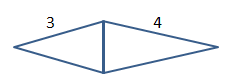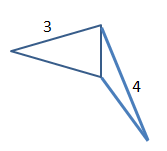## Example Questions

### Example Question #2 : Acute / Obtuse Triangles

What is the area of a triangle with side lengths 18, 24, and 30?

216

140

342

280

196

216

Explanation:

The question doesn't tell us if this is a right triangle, so we can't assume that it is. But there is a formula to find the area when we don't know the height: area = [p(p – a)(p – b)(p – c)]1/2, where a, b, and c are the side lengths and p is half of the perimeter. The perimeter is 18 + 24 + 30 = 72, so p = 72/2 = 36.

Then area = [36(36 – 18)(36 – 24)(36 – 30)]1/2 = [36 * 12 * 6 * 18]1/2 = 216.

### Example Question #3 : Acute / Obtuse Triangles

You are asked which triangle is larger. You are only told that theyhave the same base length and that one contains at least one 3 inch side and the other contains at least one 4 inch side. Determine whether the left or right triangle is larger.It is impossible to determine from the given information.

The triangles are equal

The right triangle is larger

The left triangle is larger

It is impossible to determine from the given information.

Explanation:

Since we are told nothing about the angles we cannot assume that these are isosceles triangles and are open to possibilites such as that shown below in which the left side would be larger. If both were isosceles triangles then the right side would be larger.Tired of practice problems?

Try live online GRE prep today.